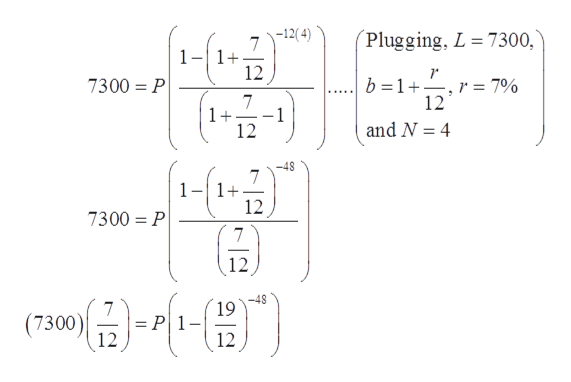# Calculus Question

Question
104 views
check_circle

Step 1

It is given that, L dollars are borrowed for N years at interest rate r per annum and monthly payment (P) is calculated using the equation

Step 2

To find:

• The value of payment (P), if L=7300, n=4 and r=7%.
• If loan L=\$7300 is paid back over four years with annual payment P=\$200, then apply Newton’s Method to compute the implied interest rate r of this loan.
Step 3
• From (1), the value of payment P can be evaluated by substitut...help_outlineImage Transcriptionclose|-12( 4) Plugging, L 7300, 7 1+ 12 r r = 7% 12 7300 P b 1 고기 7 1+ -1 12 and N 4 -48 7 1+ 12 7300 P 7 12 7 19 (7300) 12 12 fullscreen

### Want to see the full answer?

See Solution

#### Want to see this answer and more?

Solutions are written by subject experts who are available 24/7. Questions are typically answered within 1 hour.*

See Solution
*Response times may vary by subject and question.
Tagged in

### Calculus